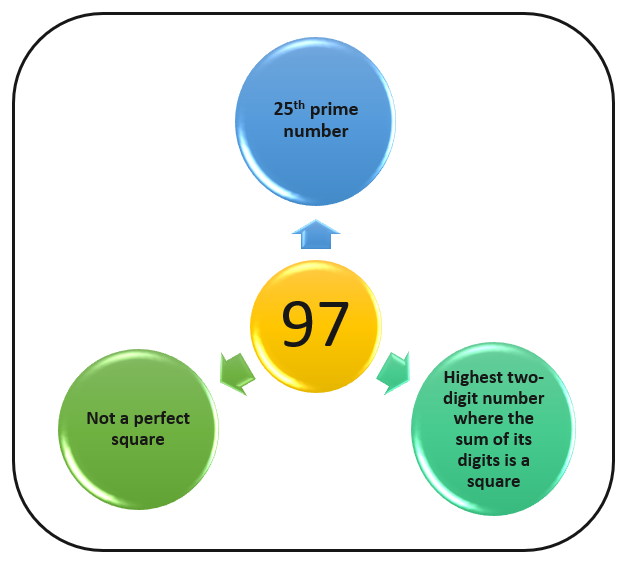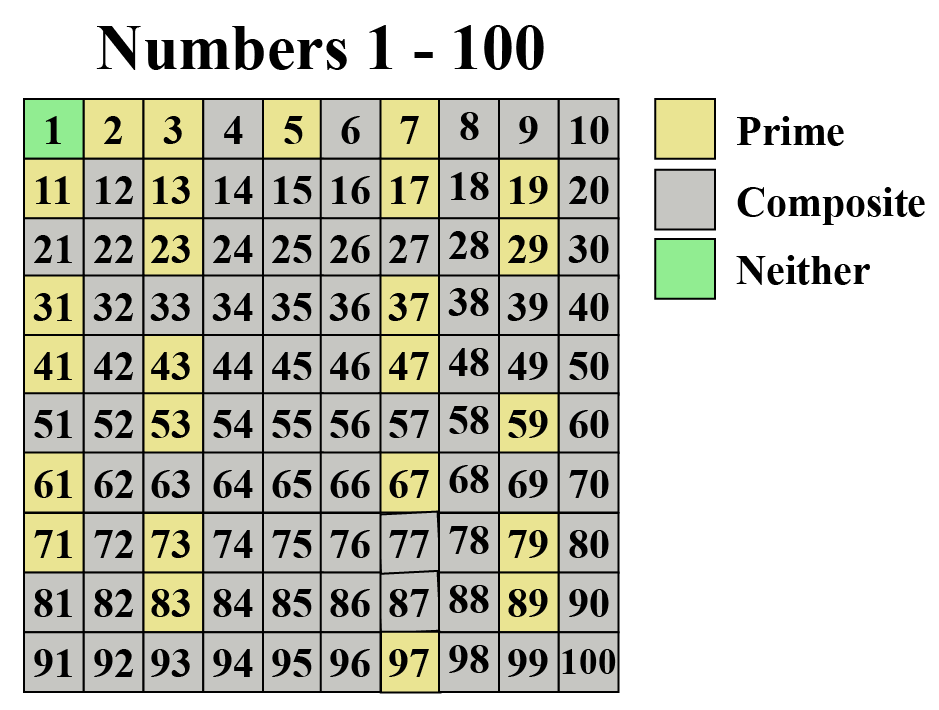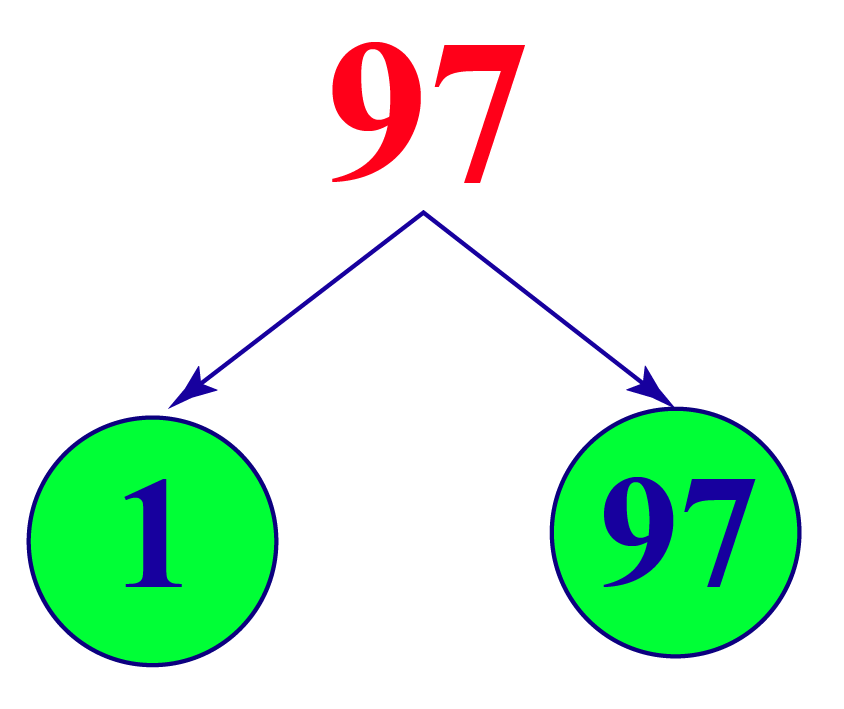# Is 97 A Prime Number?

Is 97 A Prime Number?
Go back to  'Prime Numbers'

97 is a prime number. This means it appears only in the multiplication tables of 1 and 97, No other number can divide it completely. Let us learn more about the number 97Once you understand this concept, you will also understand how it's easy to confuse prime and composite numbers. Take the example of 91 and 93. Is 91 a prime number? Or for that matter, is 93 a prime number? Initial observation may cause you to think so, but when you look at the rules of multiples of 3, you realize that both 91 and 93 are composite numbers.

Check out the interactive solved examples to know more about the lesson and try your hand at solving a few interesting practice questions at the end of the page.

## What is a Prime Number?

A whole number that cannot be written as the product of small numbers is called the prime number.

Prime numbers are non-composite numbers.

### Prime Numbers

The numbers which have only two factors either 1 or the number itself are known as prime numbers.

Prime numbers from 1 to 100 are color-coded in the picture given below.### Composite Numbers

The numbers which have more than two factors are known as composite numbers.

The numbers which are not highlighted in the above picture are composite numbers.

## Is 97 a Prime Number?

Yes, 97 is a prime number.

The number 97 has only two factors one is number 1 and the other is 97 itself.Factors of 97

No other integer number divide 97 completely, hence 97 is a prime number.

## Is 97 a Composite Number?

97 is not a composite number because 97 has only two factors 1 and 97

A number either can be a prime number or a composite number it can not be both at a time.Think Tank
• If we add all the prime numbers less than 100 then what will be the sum?
• Can the product of two prime numbers be a prime number?

## Solved Examples

 Example 1

Which number on multiplying with (-1) gives 97?

Solution

To find this, we need to divide 97 by (-1) =

$\text{97} \div \text{(-1)} = \text{-97}$

 $$\therefore$$ - 97 on multiplying with - 1 gives 97
 Example 2

Ron makes a statement that 7, 17, 37, 47, 67, and 97 are all prime numbers. Is Ron correct?

Solution

Yes, all the above-given numbers are prime numbers since they only have two factors 1 and the number itself.

So, Ron is correct.

 $$\therefore$$ 7, 17, 37, 47, 67, and 97 are all prime numbers.
 Example 3

Ashley challenges her friends and asks them to find the nearest number to 97 which is a perfect square. What is the number on her mind?

Solution

The nearest number to 97 which is a perfect square is 100

100 is a perfect square since $$10\times10=100$$.

 $$\therefore$$ 100 is the perfect square nearest to 97

## Interactive Questions on Is 97 a Prime Number

Here are a few activities for you to practice. Select/Type your answer and click the "Check Answer" button to see the result.

## Let's Summarize

The mini-lesson targeted the fascinating concept of 'is 97 a prime number'. Along with it, we also learned is '91 a prime number' and 'is 93 a prime number'. The math journey around 'is 97 a prime number' started with what a student already knows, and went on to creatively crafting a fresh concept in the young minds. Done in a way that not only it is relatable and easy to grasp, but also will stay with them forever.

At Cuemath, our team of math experts is dedicated to making learning fun for our favorite readers, the students!

Through an interactive and engaging learning-teaching-learning approach, the teachers explore all angles of a topic.

Be it worksheets, online classes, doubt sessions, or any other form of relation, it’s the logical thinking and smart learning approach that we, at Cuemath, believe in.

## 1. What is the prime factorization of 97?

97 is a prime number that has only two factors 1 and 97. It cannot be factored.

## 2. Is 97 a composite or prime?

97 is a prime number since it has only two factors 1 and 97

## 3. Is 97 divisible by any other number?

97 is a prime number hence it is divisible by only 1 and 97

More Important Topics
Numbers
Algebra
Geometry
Measurement
Money
Data
Trigonometry
Calculus